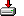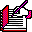#hasvar.sas```%macro hasvar (data, var, type);

%* check if a variable of a given type exists in a dataset;
%*
%* data - name of data set to check;
%* var  - name of variable in data set to check for;
%* type - type of variable in data set to check for, C, N or X;
%*
%* 0 no such variable or such data set;
%* 1 variable of indicated type exists in data set;
%*
%* Richard A. DeVenezia 08/04/99;
%* Note: if option NOTES is on and the data has a where clause
%*       that returns no rows, then there will be a note in the log
%*       NOTE: No observations were selected from data set abc.xyz.
%*       There is currently no way to circumvent this.
%* mod
%*  8/10/99 rad process type X as do not care;
%*;

%local hasvar dsid varnum vartype chektype ;

%let chektype = %upcase (%substr (&type, 1, 1));

%let hasvar = 0;

%let dsid = %sysfunc (open (&DATA));

%if &dsid %then %do;
%let varnum = %sysfunc (varnum (&dsid, &var));
%if &varnum %then %do;
%let vartype = %sysfunc (vartype (&dsid, &varnum));
%if (&vartype = &chektype) or (X = &chektype) %then
%let hasvar = 1;
%end;
%let dsid = %sysfunc (close (&dsid));
%end;

&hasvar

%mend hasvar;```